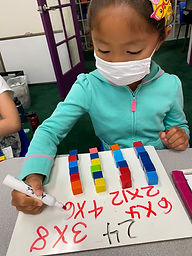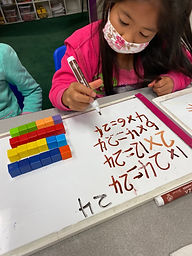## Ms. Brianne

### Target 1​

###### Lesson Type:

Continuation

Number Operation

:

Computation

Multiply within 100.

###### 1:

Understand that multiplying numbers makes numbers larger.

###### 2:

Understand that multiplication represents the combination of equal groups of objects.

###### 3:

Understand that the same quantity can be group in multiple ways, creating different multiplication equations.

###### 4:

Interpret products of whole numbers as the total number of objects arranged into equal groupings.

###### 5:

Understand that in multiplication equations, the first factor represents the number of groups and the second factor represents the quantity in each group.

3rd

###### Vocabulary:

Multiply, Times, Product, Equal groups

Activities:

• Students created different addition equations to show sums of 12. Then they circled the equations that showed equal groups and wrote the matching multiplication equations.
• Students were given the number 24 and used blocks to create equal groups in order to find a variety of multiplication equations with a product of 24.
• Students were introduced to the commutative property when comparing multiplication equations.
• Students used the commutative property to help them complete their multiplication magic squares.### Home Exploration

###### Guiding Questions:## Absent Students:

### Target 2

:

###### 1:

Compose larger shapes from smaller shapes.

###### 2:

Study geometric shapes to derive the component shapes that can be used to create it.

4th

###### Vocabulary:

2D Shapes, Composite Shape

Activities:

• Students were given task cards and used a specific amount of pattern blocks to create a composite (bigger) shape.
• Students were given an irregular outline and had to fit the pattern blocks within the space provided.### Home Exploration

###### Guiding Questions:### Target 3

:

###### Vocabulary:

Activities:### Home Exploration